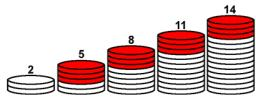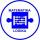# 6 terms

Find the first six terms of the sequence.

a1 = 7, an = an-1 + 6

a1 =  7
a2 =  13
a3 =  19
a4 =  25
a5 =  31
a6 =  37

### Step-by-step explanation:

${a}_{1}=7$
${a}_{2}=7+6=13$
${a}_{3}=13+6=19$
${a}_{4}=19+6=25$
${a}_{5}=25+6=31$
${a}_{6}=31+6=37$We will be pleased if You send us any improvements to this math problem. Thank you!## Related math problems and questions:

• Six termsFind the first six terms of the sequence a1 = -3, an = 2 * an-1
• Geometric progressiobIf the sum of four consective terms of geometric progression is 80 and arithmetic mean of second and fourth term is 30 then find terms?
• Nineteenth memberFind the nineteenth member of the arithmetic sequence: a1=33 d=5 find a19
• SequenceIn the arithmetic sequence is a1=-1, d=4. Which member is equal to the number 203?
• Find the 21Find the sum of the six terms of the finite geometric sequence 96, -48, 24, -12
• Sequence 2Write the first 5 members of an arithmetic sequence a11=-14, d=-1
• SequenceWrite the first 7 members of an arithmetic sequence: a1=-3, d=6.
• SequenceWrite the first 6 members of this sequence: a1 = 5 a2 = 7 an+2 = an+1 +2 an
• AP - simpleFind the first ten members of the sequence if a11 = 132, d = 3.
• Sequence 11What is the nth term of this sequence 1, 1/2, 1/3, 1/4, 1/5?
• SequenceIn the arithmetic sequence is given: Sn=2304, d=2, an=95 Calculate a1 and n.
• Arithmetic progression 2The 3rd term of an Arithmetic progression is ten more than the first term, while the fifth term is 15 more than the second term. Find the sum of the 8th and 15th terms of the Arithmetic progression if the 7th term is seven times the first term.
• Finite arithmetic sequenceHow many numbers should be inserted between the numbers 1 and 25 so that all numbers create a finite arithmetic sequence and that the sum of all members of this group is 117?
• AP - simpleDetermine the first nine elements of sequence if a10 = -1 and d = 4
• AS - sequenceWhat are the first ten members of the sequence if a11=22, d=2.
• Sequence 3Write the first 5 members of an arithmetic sequence: a4=-35, a11=-105.
• SequenceBetween numbers 1 and 53 insert n members of the arithmetic sequence that its sum is 702.